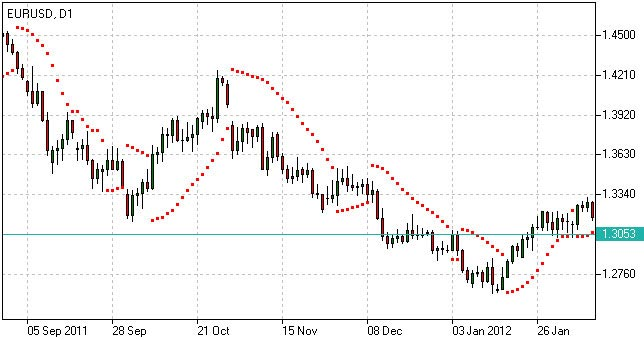# Parabolic SAR - Parabolic Indicator

`How to Use - Parabolic SAR Formula`

## Parabolic SAR Definition

Parabolic SAR is a trend following indicator developed by Welles Wilder and designed to confirm or reject trend direction, to determine trend end, correction or flat stages as well as to indicate possible exit points. The underlying principle of the indicator can be described as “stop and reverse” (SAR).

Test the Indicator in Action
Once opened Demo you will be supplied with educational materials and online support in your own language

## How to Use Parabolic SAR

When using Parabolic SAR indicator we should take into consideration its positioning against the price chart as well as its acceleration factor which increases together with the trend. Despite being a popular tool of analysis, it has limitations and may give false signals in frequently changing market conditions.

The indicator may signal the following:

Trend confirmation

• If the indicator is plotted below the price graph, it stands for an uptrend;
• If the indicator is plotted above the price graph, it confirms a downtrend.

Exit points determination

• If the price drops below Parabolic line during an uptrend, there may be sense in closing long positions;
• If the price rises above Parabolic curve during a downtrend, there may be sense in closing short positions.

Signal significance is determined with the use of the acceleration factor. The acceleration factor increases each time the close price is higher than its previous value in an uptrend and lower in a downtrend. It is believed that the indicator is more reliable when the price’s and the indicator’s moves are parallel and less reliable when they converge.Parabolic SAR Indicator

## Parabolic SAR Formula (Calculation)

To calculate the Parabolic indicator, the acceleration factor is multiplied by the difference between the low/high prices and the previous period of SAR. Then, the obtained result in case of the falling SAR is subtracted from the SAR value of the previous period, and in case of the rising SAR, added to the SAR value of the previous period.

Here is the formula for calculating the Parabolic SAR:

P(t) = P(t-1) + AF x (EP(t-1) – P(t-1))

In the Parabolic SAR formula, the P(t) is the current value of the indicator, the P(t-1) is the value in the previous period, the AF is acceleration factor, generally rising from 0.02 to 0.2 with a step of 0.02, the EP(t-1) is the extreme price in the previous period.